Author: Oscar Cronquist Article last updated on January 08, 2023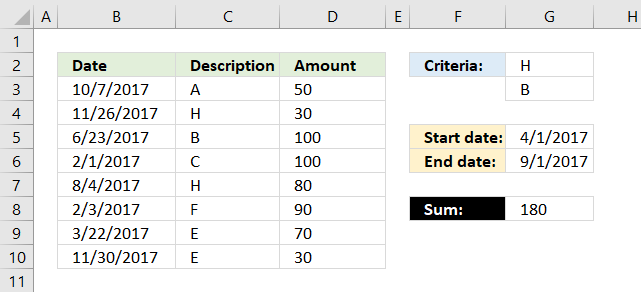## 1. SUMPRODUCT - based on a list of values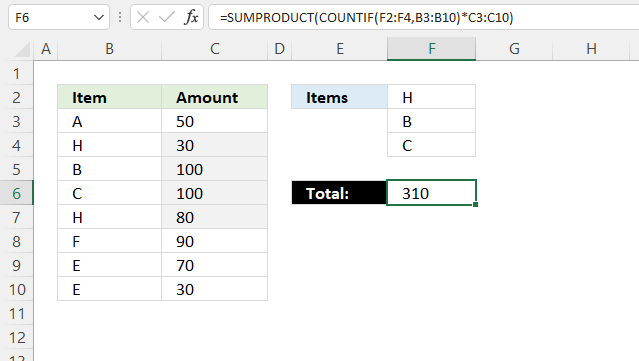The image above shows how to sum numbers in column C based on a list of conditions specified in cell range F2:F3.

If the cells in cell range B3:B10 equals any of the conditions the corresponding amount in column C is added to the total.

Check out this article if you want to apply conditions to a column each: How to use multiple conditions in the SUMPRODUCT function

Formula in cell F6:

=SUMPRODUCT(COUNTIF(F2:F4, B3:B10)*C3:C10)

### 1.1 Explaining formula in cell F6

#### Step 1 - Identify matching items

The COUNTIF function calculates the number of cells that is equal to a condition. You can also use the COUNTIF function to count cells based on multiple conditions, the result is an array containing numbers that correspond to the cell range.

COUNTIF(rangecriteria)

COUNTIF(F2:F4, B3:B10)

becomes

COUNTIF({"H"; "B"; "C"},{"A"; "H"; "B"; "C"; "H"; "F"; "E"; "E"})

and returns

{0; 1; 1; 1; 1; 0; 0; 0}. Notice that the array correspond to cell range B3:B10, values in cells F2:F4 are 1 in the array and cells not matching are 0 (zero).

#### Step 2 - Multiply array with numbers

The asterisk character lets you multiply values in Excel, it works fine with arrays as well.

COUNTIF(F2:F4, B3:B10)*C3:C10

becomes

{0; 1; 1; 1; 1; 0; 0; 0}*C3:C10

becomes

{0; 1; 1; 1; 1; 0; 0; 0}*{50; 30; 100; 100; 80; 90; 70; 30}

and returns

{0; 30; 100; 100; 80; 0; 0; 0}.

#### Step 3 - Add numbers and return a total

The SUMPRODUCT function calculates the product of corresponding values and then returns the sum of each multiplication.

SUMPRODUCT(array1, [array2], ...)

SUMPRODUCT(COUNTIF(F2:F4, B3:B10)*C3:C10)

becomes

SUMPRODUCT({0; 30; 100; 100; 80; 0; 0; 0})

and returns 310 in cell F6.

## 2. SUMPRODUCT - multiple criteriaThe formula above in cell G8 uses two conditions in cell G2 and G3 and a date range G5:G6 to create a total. The conditions in cell G2 and G3 are matched to the "Description" values in column C and the date range specified in cell G5 and G6 are used to filter values based on the dates in column B.

=SUMPRODUCT(COUNTIF(G2:G3,C3:C10)* (G5<=B3:B10)* (G6>=B3:B10)* D3:D10)

### 2.1 Explaining formula in cell G8

#### Step 1 - Identify values matching cell G2 and G3

The COUNTIF function allows you to compare multiple values to one column.

COUNTIF(G2:G3, C3:C10)

becomes

COUNTIF({"H";"B"},{"A";"H";"B";"C";"H";"F";"E";"E"})

and returns

{0;1;1;0;1;0;0;0}.

This tells us that the second, third and fifth value in column C matches G2 or G3.

The picture below shows the array in column A.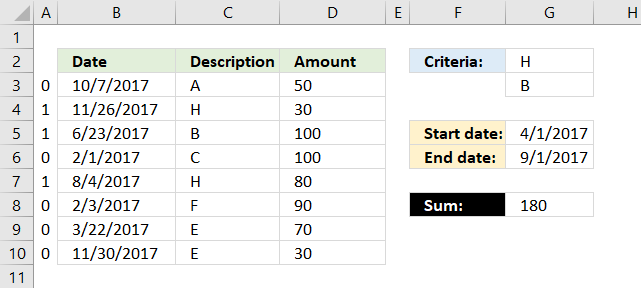#### Step 2 - Identify values matching the date range

To match a date range we need two logical expressions, the first one checks if any of the dates in B3:B10 are larger than or equal to the start date in G5.

(G5<=B3:B10)

returns

{TRUE; TRUE; TRUE; FALSE; TRUE; FALSE; FALSE; TRUE}.

The second logical expression matches dates smaller than or equal to the end date.

(G6>=B3:B10)

returns

{FALSE; FALSE; TRUE; TRUE; TRUE; TRUE; TRUE; FALSE}

#### Step 3 - Apply AND logic to all arrays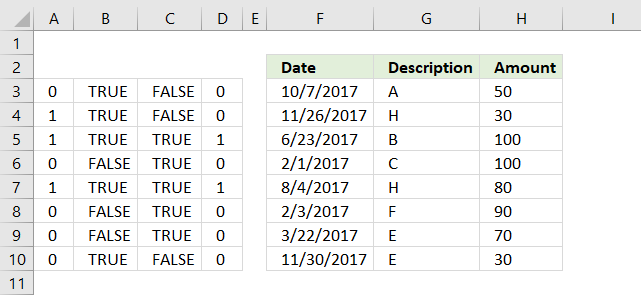Column D shows the result of multiplying all arrays from the logical expressions and the COUNTIF function. This is the same as AND logic for values row-wise.

For example, the first value on row 3 is 0 (zero), the next is TRUE and the last value is FALSE. 0*TRUE*FALSE equals FALSE. All values must be TRUE to return TRUE.

Note, 0 (zero) is the same as FALSE and 1 is TRUE in Excel. Row 5 and 7 in column D return 1.

#### Step 4 - Sum all numbers in the array

The SUMPRODUCT function creates a total based on logical expressions.

SUMPRODUCT(COUNTIF(G2:G3,C3:C10)* (G5<=B3:B10)* (G6>=B3:B10)* D3:D10)

becomes

SUMPRODUCT({0;0;1;0;1;0;0;0}* D3:D10)

Lastly, multiply the array with the amounts in column D.

SUMPRODUCT({0;0;1;0;1;0;0;0}* {50; 30; 100; 100; 80; 90; 70; 30})

calculates to

SUMPRODUCT({0;0;100;0;80;0;0;0})

and returns 180 in cell G8.## 3. SUMPRODUCT - multiple date ranges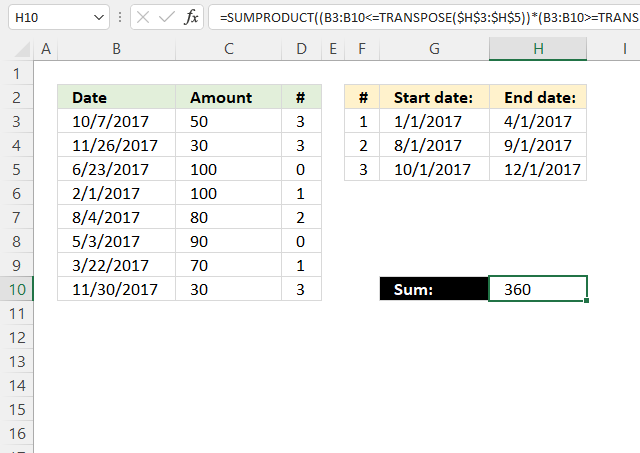The following formula adds numbers based on date ranges, if the corresponding Excel date matches a date range the number is added to the total.

For example, cell B3 contains the date 10/7/2017 which matches the third date range. 10/7/2017 is earlier than or equal to 12/1/2017 and later than or equal to 10/1/2017.

The formula in cell H10 does this comparison to all dates in B3:B10 against the date ranges specified in G3:H5 and if they match the corresponding number in D3:D10 is added to a total.

Cell range D3:D10 contains which date range the date matches, cell D3 contains 3 and the corresponding date in B3 matches date range 3 10/1/2017 - 12/1/2017.

Formula in cell H10:

=SUMPRODUCT((B3:B10<=TRANSPOSE(H3:H5))*(B3:B10>=TRANSPOSE(G3:G5))*C3:C10)

### 3.1 Explaining formula in cell H10

#### Step 1 - Transpose values

The TRANSPOSE function rearranges values from horizontal to vertical or vice versa.

TRANSPOSE(array)

TRANSPOSE(H3:H5)

becomes

TRANSPOSE({42826; 42979; 43070})

and returns {42826, 42979, 43070}.

Notice how the delimiting characters change, the semicolon is converted to a comma. You may see other characters, they are based on your regional settings.

#### Step 2 - Compare dates to end date ranges

The less than and equal sign combined lets you compare dates in B3:B10 to H3:H5, the result is either TRUE or FALSE.

B3:B10<=TRANSPOSE(H3:H5)

becomes

B3:B10<={42826, 42979, 43070}

becomes

{43015; 43065; 42909; 42767; 42951; 42858; 42816; 43069}<={42826, 42979, 43070}

and returns

{FALSE, FALSE, TRUE; FALSE, FALSE, TRUE; FALSE, TRUE, TRUE; TRUE, TRUE, TRUE; FALSE, TRUE, TRUE; FALSE, TRUE, TRUE; TRUE, TRUE, TRUE; FALSE, FALSE, TRUE}.

Notice that the delimiting character is ; in the first array and , in the second array. This will compare vertical dates to horizontal dates and the result is a table.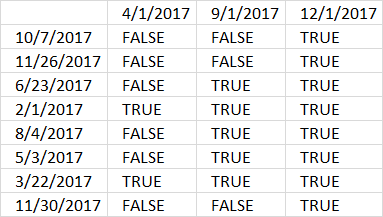#### Step 3 - Compare dates to start date ranges

We can use the exact same technique described above in step 2 to compare dates with start dates.

B3:B10>=TRANSPOSE(\$G\$3:\$G\$5)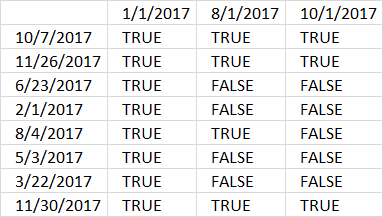The horizontal dates are now start dates.

#### Step 4 - Multiply arrays

Both calculated boolean values must be TRUE to match a date range, we can use the asterisk character to multiply the arrays.

The result is always 1 or 0 (zero) when you multiply boolean values. 1 is the numerical equivalent to TRUE and 0 (zero) is FALSE.

(B3:B10<=TRANSPOSE(H3:H5))*(B3:B10>=TRANSPOSE(G3:G5))

The parentheses let you control the order of calculations, we must multiply after the comparisons are made.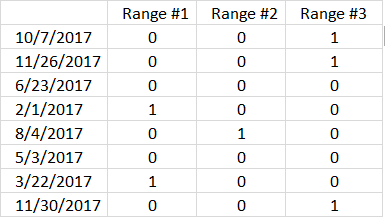#### Step 5 - Multiply array with numbers

We now know which range each date belongs to, however, you may have noticed that some dates have no date range.

(B3:B10<=TRANSPOSE(H3:H5))*(B3:B10>=TRANSPOSE(G3:G5))*C3:C10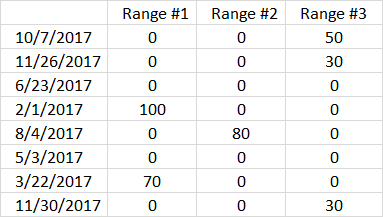#### Step 6 - Sum numbers in array

The SUMPRODUCT function adds the numbers and returns a total.

SUMPRODUCT((B3:B10<=TRANSPOSE(H3:H5))*(B3:B10>=TRANSPOSE(G3:G5))*C3:C10)

becomes

SUMPRODUCT({0, 0, 50; 0, 0, 30; 0, 0, 0; 100, 0, 0; 0, 80, 0; 0, 0, 0; 70, 0, 0; 0, 0, 30})

and returns 360 in cell H10.The following formula returns the corresponding date range number, this lets you quickly note which date range a date belongs to.

Formula in cell D3:

=SUMPRODUCT((B3<=\$H\$3:\$H\$5)*(B3>=\$G\$3:\$G\$5)*\$F\$3:\$F\$5)

## 4. SUMPRODUCT - sum based on currency rates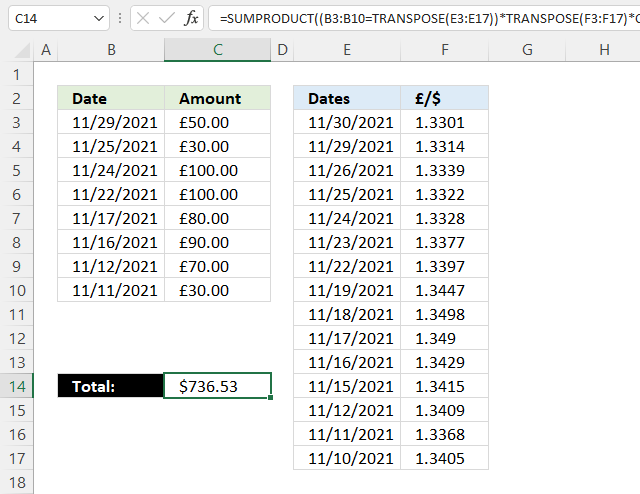Formula in cell C14:

=SUMPRODUCT((B3:B10=TRANSPOSE(E3:E17))*TRANSPOSE(F3:F17)*C3:C10)

### 4.1 Explaining formula

#### Step 1 - Rearrange values

The TRANSPOSE function rearranges values from horizontal to vertical or vice versa.

TRANSPOSE(array)

TRANSPOSE(E3:E17)

becomes

TRANSPOSE({44530; 44529; 44526; 44525; 44524; 44523; 44522; 44519; 44518; 44517; 44516; 44515; 44512; 44511; 44510})

and returns

{44530, 44529, 44526, 44525, 44524, 44523, 44522, 44519, 44518, 44517, 44516, 44515, 44512, 44511, 44510}.

#### Step 2 - Compare values

The equal sign lets you compare values, TRUE if identical (not case sensitive) and FALSE if not.

B3:B10=TRANSPOSE(E3:E17)

becomes

B3:B10={44530, 44529, 44526, 44525, 44524, 44523, 44522, 44519, 44518, 44517, 44516, 44515, 44512, 44511, 44510}

becomes

{44529; 44525; 44524; 44522; 44517; 44516;44512;44511}={44530, 44529, 44526, 44525, 44524, 44523, 44522, 44519, 44518, 44517, 44516, 44515, 44512, 44511, 44510}

and returns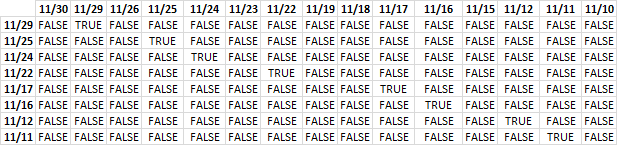The image above shows the array containing the boolean values TRUE or FALSE, currency dates are above the array arranged horizontally. The other dates are arranged vertically and located to the left of the array.

#### Step 3 - Multiply with currency data

(B3:B10=TRANSPOSE(E3:E17))*TRANSPOSE(F3:F17)

returns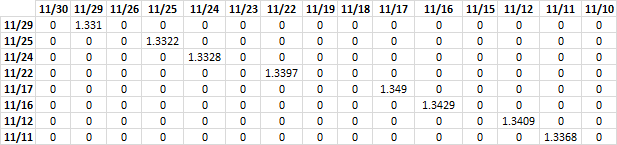FALSE * currency_data = 0 (zero)
TRUE * currency_data = currency_data

The array contains the corresponding currency rate if the dates match. If dates don't match 0 (zero) is returned.

#### Step 4 - Multiply with amounts

(B3:B10=TRANSPOSE(E3:E17))*TRANSPOSE(F3:F17)*C3:C10

returns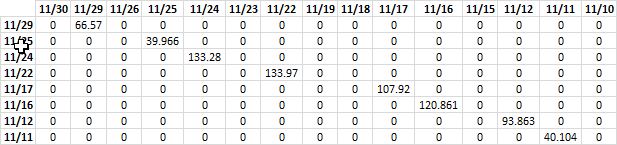#### Step 5 - Sum numbers

The SUMPRODUCT function adds the numbers and returns a total.

SUMPRODUCT((B3:B10=TRANSPOSE(E3:E17))*TRANSPOSE(F3:F17)*C3:C10)

becomes

SUMPRODUCT({0, 66.57, 0, 0, 0, 0, 0, 0, 0, 0, 0, 0, 0, 0, 0;0, 0, 0, 39.966, 0, 0, 0, 0, 0, 0, 0, 0, 0, 0, 0;0, 0, 0, 0, 133.28, 0, 0, 0, 0, 0, 0, 0, 0, 0, 0;0, 0, 0, 0, 0, 0, 133.97, 0, 0, 0, 0, 0, 0, 0, 0;0, 0, 0, 0, 0, 0, 0, 0, 0, 107.92, 0, 0, 0, 0, 0;0, 0, 0, 0, 0, 0, 0, 0, 0, 0, 120.861, 0, 0, 0, 0;0, 0, 0, 0, 0, 0, 0, 0, 0, 0, 0, 0, 93.863, 0, 0;0, 0, 0, 0, 0, 0, 0, 0, 0, 0, 0, 0, 0, 40.104, 0})

and returns 736.53 in cell C14.## 5. Get Excel *.xlsx

### Get the Excel fileSUMPRODUCT-multiple-criteriav2.xlsx